Chapter 6, Problem 6.7EP### Organic And Biological Chemistry

7th Edition
STOKER + 1 other
ISBN: 9781305081079

#### Solutions

Chapter
Section### Organic And Biological Chemistry

7th Edition
STOKER + 1 other
ISBN: 9781305081079
Textbook Problem

# Indicate whether or not each of the following compounds contains an amine functional group?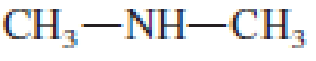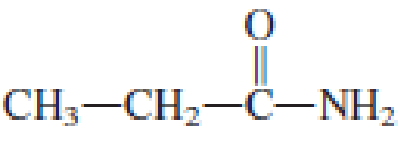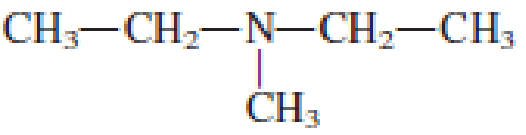(a)

Interpretation Introduction

Interpretation:

The given compound contains an amine functional group or not has to be indicated.

Concept Introduction:

Amine is an organic derivative.  If in ammonia one or more alkyl, cycloalkyl, or aryl groups are substituted instead of hydrogen atom then it is known as amine.  Depending on the number of substitution the amines are classified as primary, secondary or tertiary amine.  Primary amine is the one in which only one hydrogen atom in ammonia is replaced by a hydrocarbon group.  Secondary amine is the one in which only two hydrogen atoms in ammonia is replaced by a hydrocarbon group.  Tertiary amine is the one in which all three hydrogen atoms in ammonia is replaced by a hydrocarbon group.  The generalized structural formula for all the amines is,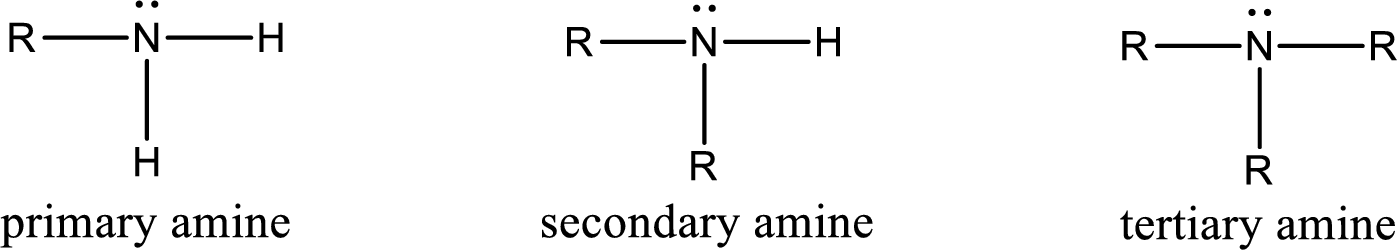Amides are also organic derivative.  In an amide, the nitrogen atom is bonded to a carbonyl group.  The general structural formula of amide can be given as shown below,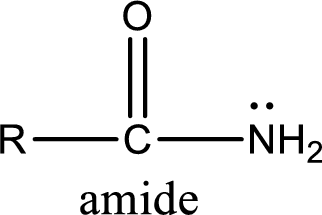The difference between amine and amide is that in amine, the nitrogen atom is bonded to a hydrocarbon chain.  In case of amides, the nitrogen atom is bonded to a carbonyl group.

Explanation

Given organic compound is,

On looking into the above structure, it is found that a nitrogen atom is directly bonded to a hydrocarbon group.  Hence, this compound contains amine functional group...

(b)

Interpretation Introduction

Interpretation:

The given compound contains an amine functional group or not has to be indicated.

Concept Introduction:

Amine is an organic derivative.  If in ammonia one or more alkyl, cycloalkyl, or aryl groups are substituted instead of hydrogen atom then it is known as amine.  Depending on the number of substitution the amines are classified as primary, secondary or tertiary amine.  Primary amine is the one in which only one hydrogen atom in ammonia is replaced by a hydrocarbon group.  Secondary amine is the one in which only two hydrogen atoms in ammonia is replaced by a hydrocarbon group.  Tertiary amine is the one in which all three hydrogen atoms in ammonia is replaced by a hydrocarbon group.  The generalized structural formula for all the amines is,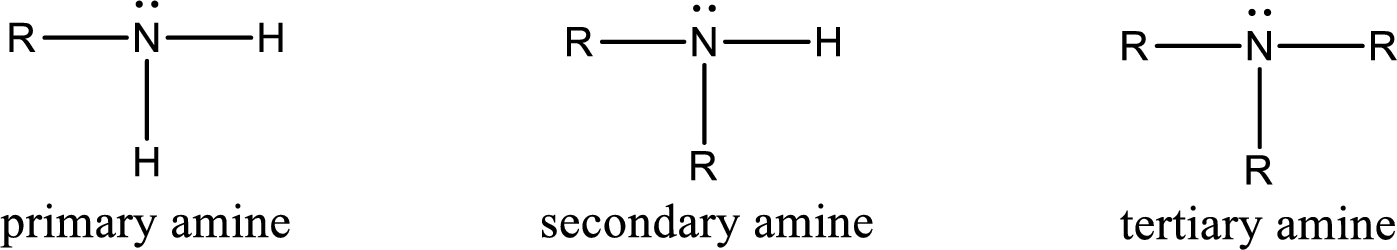Amides are also organic derivative.  In an amide, the nitrogen atom is bonded to a carbonyl group.  The general structural formula of amide can be given as shown below,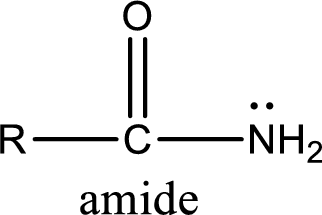The difference between amine and amide is that in amine, the nitrogen atom is bonded to a hydrocarbon chain.  In case of amides, the nitrogen atom is bonded to a carbonyl group.

(c)

Interpretation Introduction

Interpretation:

The given compound contains an amine functional group or not has to be indicated.

Concept Introduction:

Amine is an organic derivative.  If in ammonia one or more alkyl, cycloalkyl, or aryl groups are substituted instead of hydrogen atom then it is known as amine.  Depending on the number of substitution the amines are classified as primary, secondary or tertiary amine.  Primary amine is the one in which only one hydrogen atom in ammonia is replaced by a hydrocarbon group.  Secondary amine is the one in which only two hydrogen atoms in ammonia is replaced by a hydrocarbon group.  Tertiary amine is the one in which all three hydrogen atoms in ammonia is replaced by a hydrocarbon group.  The generalized structural formula for all the amines is,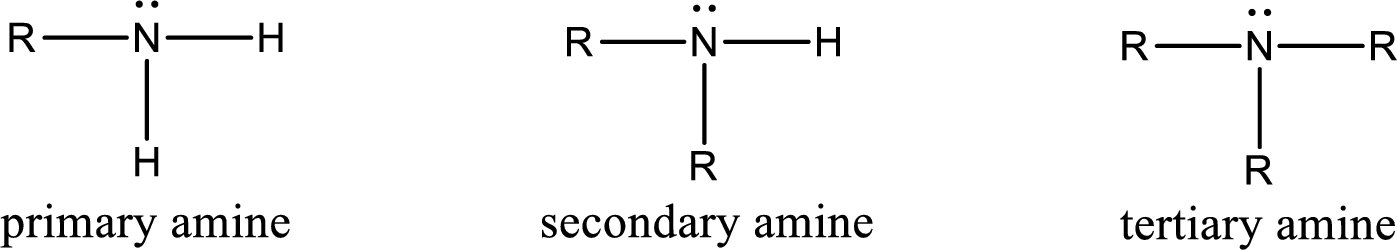Amides are also organic derivative.  In an amide, the nitrogen atom is bonded to a carbonyl group.  The general structural formula of amide can be given as shown below,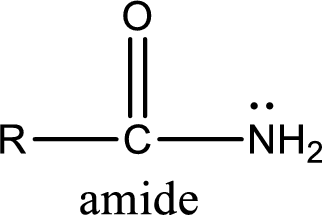The difference between amine and amide is that in amine, the nitrogen atom is bonded to a hydrocarbon chain.  In case of amides, the nitrogen atom is bonded to a carbonyl group.

(d)

Interpretation Introduction

Interpretation:

The given compound contains an amine functional group or not has to be indicated.

Concept Introduction:

Amine is an organic derivative.  If in ammonia one or more alkyl, cycloalkyl, or aryl groups are substituted instead of hydrogen atom then it is known as amine.  Depending on the number of substitution the amines are classified as primary, secondary or tertiary amine.  Primary amine is the one in which only one hydrogen atom in ammonia is replaced by a hydrocarbon group.  Secondary amine is the one in which only two hydrogen atoms in ammonia is replaced by a hydrocarbon group.  Tertiary amine is the one in which all three hydrogen atoms in ammonia is replaced by a hydrocarbon group.  The generalized structural formula for all the amines is,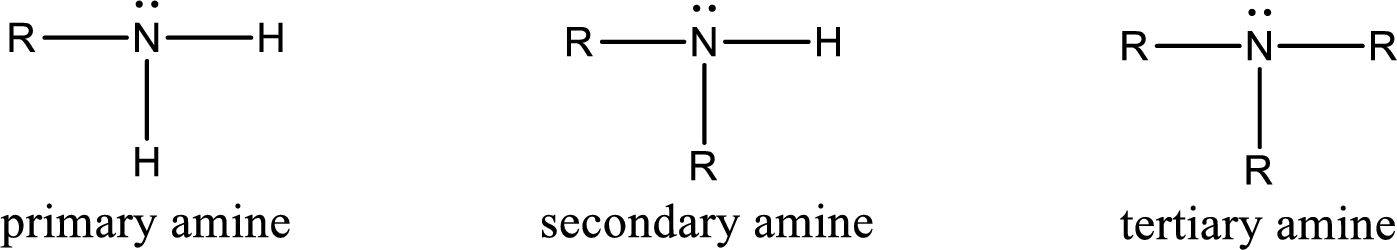Amides are also organic derivative.  In an amide, the nitrogen atom is bonded to a carbonyl group.  The general structural formula of amide can be given as shown below,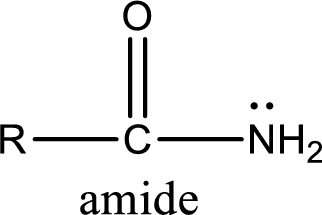The difference between amine and amide is that in amine, the nitrogen atom is bonded to a hydrocarbon chain.  In case of amides, the nitrogen atom is bonded to a carbonyl group.

### Still sussing out bartleby?

Check out a sample textbook solution.

See a sample solution

#### The Solution to Your Study Problems

Bartleby provides explanations to thousands of textbook problems written by our experts, many with advanced degrees!

Get Started